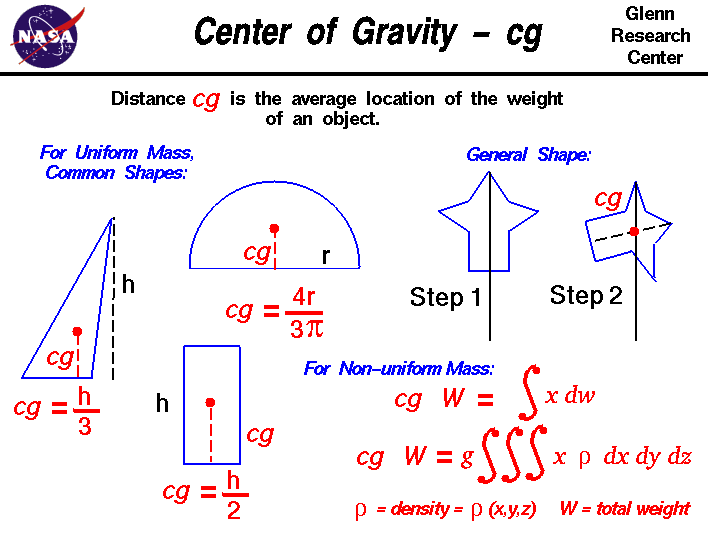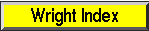+ Text Only Site
+ Non-Flash Version
+ Contact GlennThe center of gravity is a geometric property of any object. The center of gravity is the average location of the weight of an object. We can completely describe the motion of any object through space in terms of the translation of the center of gravity of the object from one place to another, and the rotation of the object about its center of gravity if it is free to rotate. If the object is confined to rotate about some other point, like a hinge, we can still describe its motion. In flight, both airplanes and rockets rotate about their centers of gravity. A kite, on the other hand, rotates about the bridle point. But the trim of a kite still depends on the location of the center of gravity relative to the bridle point, because for every object the weight always acts through the center of gravity. Determining the center of gravity is very important for any flying object. How do engineers determine the location of the center of gravity for an aircraft which they are designing? In general, determining the center of gravity (cg) is a complicated procedure because the mass (and weight) may not be uniformly distributed throughout the object. The general case requires the use of calculus which we will discuss at the bottom of this page. If the mass is uniformly distributed, the problem is greatly simplified. If the object has a line (or plane) of symmetry, the cg lies on the line of symmetry. For a solid block of uniform material, the center of gravity is simply at the average location of the physical dimensions. (For a rectangular block, 50 X 20 X 10, the center of gravity is at the point (25,10, 5) ). For a triangle of height h, the cg is at h/3, and for a semi-circle of radius r, the cg is at (4*r/(3*pi)) where pi is ratio of the circumference of the circle to the diameter. There are tables of the location of the center of gravity for many simple shapes in math and science books. The tables were generated by using the equation from calculus shown on the slide. For a general shaped object, there is a simple mechanical way to determine the center of gravity: If we just balance the object using a string or an edge, the point at which the object is balanced is the center of gravity. (Just like balancing a pencil on your finger!) Another, more complicated way, is a two step method shown on the slide. In Step 1, you hang the object from any point and you drop a weighted string from the same point. Draw a line on the object along the string. For Step 2, repeat the procedure from another point on the object You now have two lines drawn on the object which intersect. The center of gravity is the point where the lines intersect. This procedure works well for irregularly shaped objects that are hard to balance. If the mass of the object is not uniformly distributed, we must use calculus to determine center of gravity. We will use the symbol S dw to denote the integration of a continuous function with respect to weight. Then the center of gravity can be determined from: cg * W = S x dw where x is the distance from a reference line, dw is an increment of weight, and W is the total weight of the object. To evaluate the right side, we have to determine how the weight varies geometrically. From the weight equation, we know that: w = m * g where m is the mass of the object, and g is the gravitational constant. In turn, the mass m of any object is equal to the density, rho, of the object times the volume, V: m = rho * V We can combine the last two equations: w = g * rho * V then dw = g * rho * dV dw = g * rho(x,y,z) * dx dy dz If we have a functional form for the mass distribution, we can solve the equation for the center of gravity: cg * W = g * SSS x * rho(x,y,z) dx dy dz where SSS indicates a triple integral over dx. dy. and dz. If we don't know the functional form of the mass distribution, we can numerically integrate the equation using a spreadsheet. Divide the distance into a number of small volume segments and determining the average value of the weight/volume (density times gravity) over that small segment. Taking the sum of the average value of the weight/volume times the distance times the volume segment divided by the weight will produce the center of gravity. You can view a short movie of "Orville and Wilbur Wright" explaining how the center of gravity affected the flight of their aircraft. The movie file can be saved to your computer and viewed as a Podcast on your podcast player. Activities:Guided ToursAircraft Weight:Fuselage:Navigation ..Beginner's Guide Home Page+ Inspector General Hotline + Equal Employment Opportunity Data Posted Pursuant to the No Fear Act + Budgets, Strategic Plans and Accountability Reports + Freedom of Information Act + The President's Management Agenda + NASA Privacy Statement, Disclaimer, and Accessibility CertificationEditor: Nancy Hall NASA Official: Nancy Hall Last Updated: May 05 2015 + Contact Glenn